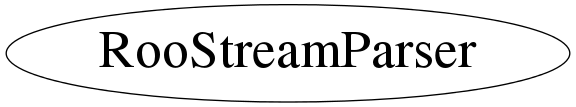# class RooStreamParser

```
RooStreamParser is a utility class to parse istreams into tokens and optionally
convert them into basic types (double,int,string)

The general tokenizing philosophy is that there are two kinds of tokens: value
and punctuation. The former are variable length, the latter always
one character. A token is terminated if one of the following conditions
occur
- space character found (' ',tab,newline)
- change of token type (value -> punctuation or vv)
- end of fixed-length token (punctuation only)
- start or end of quoted string

The parser is aware of floating point notation and will assign leading
minus signs, decimal points etc to a value token when this is obvious
from the context. The definition of what is punctuation can be redefined.

```

## Function Members (Methods)

public:
 virtual ~RooStreamParser() Bool_t atEOF() Bool_t atEOL() static TClass* Class() Bool_t convertToDouble(const TString& token, Double_t& value) Bool_t convertToInteger(const TString& token, Int_t& value) Bool_t convertToString(const TString& token, TString& string) Bool_t expectToken(const TString& expected, Bool_t zapOnError = kFALSE) TString getPunctuation() const virtual TClass* IsA() const Bool_t isPunctChar(char c) const RooStreamParser& operator=(const RooStreamParser&) Bool_t readDouble(Double_t& value, Bool_t zapOnError = kFALSE) Bool_t readInteger(Int_t& value, Bool_t zapOnError = kFALSE) TString readLine() Bool_t readString(TString& value, Bool_t zapOnError = kFALSE) TString readToken() RooStreamParser(istream& is) RooStreamParser(const RooStreamParser&) RooStreamParser(istream& is, const TString& errPrefix) void setPunctuation(const TString& punct) virtual void ShowMembers(TMemberInspector& insp) const virtual void Streamer(TBuffer&) void StreamerNVirtual(TBuffer& ClassDef_StreamerNVirtual_b) void zapToEnd(Bool_t inclContLines = kFALSE)

## Data Members

protected:
 Bool_t _atEOF Bool_t _atEOL istream* _is TString _prefix TString _punct

## Class Charts## Function documentation

RooStreamParser(istream& is)
``` Construct parser on given input stream
```
RooStreamParser(istream& is, const TString& errPrefix)
``` Construct parser on given input stream. Use given errorPrefix to
prefix any parsing error messages
```

``` Destructor
```

``` If true, parser is at end of line in stream
```
void setPunctuation(const TString& punct)
``` Change list of characters interpreted as punctuation
```
Bool_t isPunctChar(char c) const
``` Check if given char is considered punctuation
```

``` Read one token separated by any of the know punctuation characters
This function recognizes and handles comment lines in the istream (those
starting with '#', quoted strings ("") the content of which is not tokenized
and '+-.' characters that are part of a floating point numbers and are exempt
from being interpreted as a token separator in case '+-.' are defined as
token separators.
```

``` Read an entire line from the stream and return as TString
This method recognizes the use of '\\' in the istream
as line continuation token.
```
void zapToEnd(Bool_t inclContLines = kFALSE)
``` Eat all characters up to and including then end of the
current line. If inclContLines is kTRUE, all continuation lines
marked by the '\\' token are zapped as well
```
Bool_t expectToken(const TString& expected, Bool_t zapOnError = kFALSE)
``` Read the next token and return kTRUE if it is identical to the given 'expected' token.
```
Bool_t readDouble(Double_t& value, Bool_t zapOnError = kFALSE)
``` Read the next token and convert it to a Double_t. Returns true
if an error occurred in reading or conversion
```
Bool_t convertToDouble(const TString& token, Double_t& value)
``` Convert given string to a double. Return true if the conversion fails.
```
Bool_t readInteger(Int_t& value, Bool_t zapOnError = kFALSE)
``` Read a token and convert it to an Int_t. Returns true
if an error occurred in reading or conversion
```
Bool_t convertToInteger(const TString& token, Int_t& value)
``` Convert given string to an Int_t. Returns true if an error
occurred in conversion
```
Bool_t readString(TString& value, Bool_t zapOnError = kFALSE)
``` Read a string token. Returns true if an error occurred in reading
or conversion.  If a the read token is enclosed in quotation
marks those are stripped in the returned value
```
Bool_t convertToString(const TString& token, TString& string)
``` Convert given token to a string (i.e. remove eventual quotation marks)
```
RooStreamParser(istream& is)
``` Constructors, assignment etc.
```
TString getPunctuation() const
`{ return _punct ; }`

`{ return _atEOF ; }`